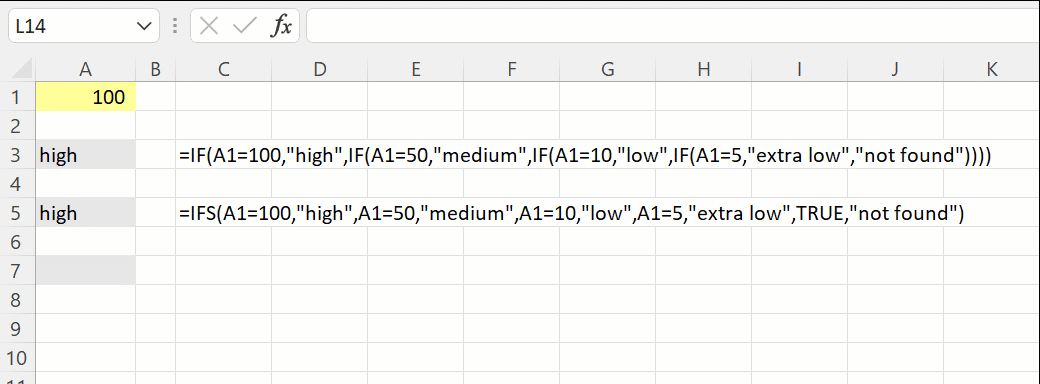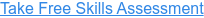Back to Blog# How to use Excel's SWITCH Function

Published
Sep 27, 2022 11:17:53 AM

Compares values against a given expression and returns the corresponding result value

#### What does Excel's SWITCH function do?

The SWITCH function is one of Excel's logical functions.

The SWITCH function evaluates an expression against a given value and returns the corresponding result value that you have provided.

The SWITCH function is an alternative to the IF and IFS functions.

An important difference between these functions is that SWITCH can only perform exact matching (ie "is equal to") it cannot perform greater than or lower than checks.

#### Here's a simple example

Let's take a look at a simple example of the SWITCH function.

We have the following business logic:

• If the value is =100 then return high
• If the value is =50 then return medium
• If the value is =10 then return low
• If the value is =5 then return extra low
• If the value is not one of the above then return "not found"Using a nested IF statement this would be structured as follows:

Using the IFS function this becomes:

Using the SWITCH function this becomes:

Notice the difference between how the three functions are structured.

#### What does that mean in plain English?

If the value in cell A1 is equal to 100, then return “High”, otherwise if it is equal to 50, then return “medium”, otherwise if it is equal to 10, then return “low” otherwise if it is equal to 5, then return “ extra low” otherwise return "not found".

#### =SWITCH(Expression, Value 1, Result 1, [Value 2], [Result 2]...)

The logical tests are in pairs: The value and corresponding result:

Expression - This is the value to match against

Value 1 – The first value to check against

Result 1 – What to return if the expression matches value 1

[Value 2] – The second value to check agains

[Result 2] – What to return if the expression matches value 2

...etc...

[Default] - Optional value to return if the expression does not match any of the given values

#### What to consider when using the SWITCH function in your financial model

• The SWITCH function allows you to test up to 126 pairs of conditions.
• The SWITCH function was introduced in 2019, so be aware of compatibility issues for users of previous Excel versions.
• XLOOKUP, VLOOKUP or INDEX MATCH can often provide a clearer and simpler solution than the SWITCH function.

Read more about the SWITCH function on the Microsoft support page here.

#### Take our free 30-question skills assessment

See where you stand with your Excel, financial modelling, data visualisation & analytics, and modelling tech skills